# Defining New Rules in the Rule Set

## Add a New Rule

To add a new rule:
1. Open the rule set where you want to add the new rule.
2. Select the Rules page. The default view on the Rules page is the horizontal view. See Selecting the Rule Set Editor View for information about the vertical and list views
3. Click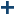.
4. Add any additional terms that the new rule requires, and add expressions for the new rule in the table cells in the rule editor. See Define Expressions for a Rule for more information.
Note: You can add up to 200 condition terms and 200 action terms to a rule set. A greater number of terms might affect performance.
5. (Optional) Change the order of the new rule. The rule order, in addition to the IF, ELSE, or OR keyword , controls how rules are evaluated within the rule set.
Tip
You can also change the order of the rules later by right-clicking on a rule and selecting either Reorder or Swap. See Change the Order of Rules in a Rule Set for more information.
6. (Optional) Modify the name of the new rule on the Rule Details tab. Rule names are limited to 100 characters and must be unique within a rule set.
7. (Optional) Clear the Record rule-fired data check box on the Rule Details tab if you do not want a rule-fired record to be written each time this rule fires.
8. (Optional) Enter a description for the new rule on the Rule Details tab.
9. Click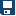.

## Selecting the Rule Set Editor View

There are three views available in the rule set editor: horizontal, vertical, and list. The default view is the horizontal view.
In the horizontal view, the terms used by the rules are displayed across the top of the rule set editor, and there is one row for each rule. You can return to the horizontal view from the other views by clicking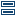.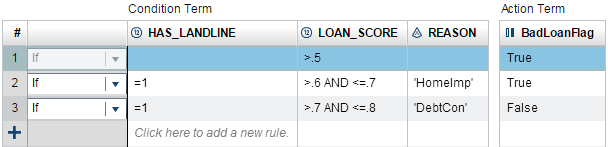To switch to the vertical view, click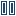. In the vertical view, the terms used by the rules are displayed in the left column, and there is one column for each rule.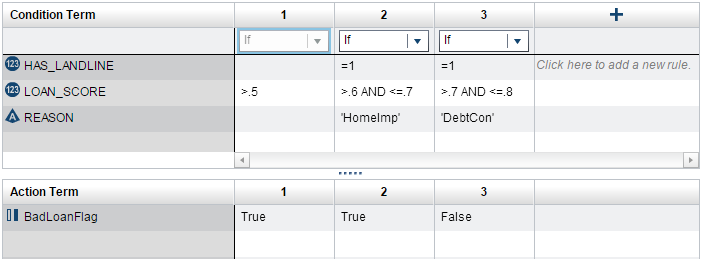To switch to the list view, click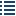. The list view is a simplified list of rules with operators, condition terms, action terms, and term values in one vertical list. By default, the rules are collapsed. Click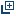to display the logic for all of the rules.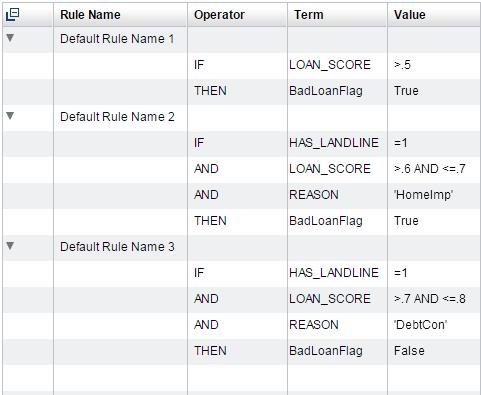Empty expressions are hidden in the list view. If you change back to the horizontal or vertical view, the empty expressions are re-displayed.
If you switch to the list view from the horizontal or vertical views, OR operators are changed to ELSE operators. You cannot select the OR operator in the list view. If you change back to the horizontal or vertical view, the OR operators remain set to ELSE operators, and every ELSE rule is assigned the appropriate action expressions.
For example, Rule Set That Defines Eight Rules in Two IF Blocks shows a rule set with OR operators in the horizontal view. The following figure shows the first IF block of the same rule set in the list view.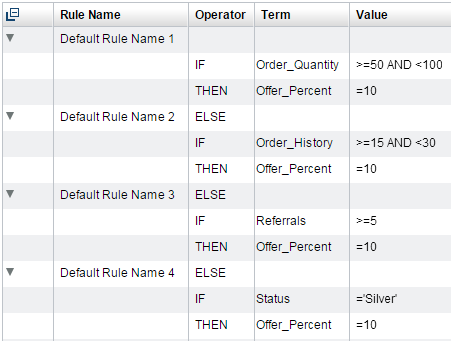## Define Expressions for a Rule

To define the expressions for a rule:
1. Add any additional terms to the rule set editor that the rule requires. For example, if your rule is `If balance < 100 then risk = "high"`, then add `balance` as the condition term and `risk` as the action term.
To add terms to the rule set, select the terms in the Vocabularies pane, and then right-click and select either Use as condition term or Use as action term. You can also drag the terms onto the rule set editor.
• To select a consecutive set of terms, click on the first term, hold down the Shift key, and click on the last term. To select nonconsecutive terms, hold down the Ctrl key, and click on each term that you want to select.
• If the terms that you want to add to the table all belong to the same entity, you can add the entire entity to the table. Note that an entity can contain many terms, and you cannot undo this operation after you have added the terms. In the horizontal and vertical views, you must delete terms one at a time. In the list view, you can select the rows containing the terms, and then right-click and select Delete. The expression for the terms is removed, and rows without expressions are hidden in the list.
In the horizontal and vertical views, terms are added to the column and row headings. In the list view, terms are added to the currently selected rule, so you must select the rule where you want to add a term before adding it.
Tip
By default, terms are displayed in the rule set editor in the same order in which they appear in the Vocabularies pane. You can reorder the terms by dragging the terms in the column or row headings.
Tip
You can add new entities and terms by clicking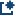in the Vocabularies pane. You can also edit existing entities and terms by right-clicking on the entity or term and selecting the appropriate option. See Create an Entity and Create a Term for more information.
2. (Optional) Select the operator for the rule. The default operator is IF. See Controlling Which Conditions Are Evaluated for more information.
3. For each term that is used in the new rule, specify the expression that applies to that term in the row or column for the new rule. For example, if the rule is `If balance < 100 then risk = "high"`, the expression for `balance` is ```< 100```, and the expression for `risk` is ```= "high"```.
Expressions can be up to 1024 characters long. They can contain numeric constants, character strings, vocabulary terms, operators, and SAS DS2 functions.
Condition expressions can contain the LOOKUP function, and action expressions can contain the LOOKUPVALUE function. However, if the expression contains the LOOKUP or LOOKUPVALUE function, then the expression cannot contain anything else.
You can enter expressions directly into the decision table, or you can use the Equation Editor to create and edit expressions. To open the Equation Editor, click in the table cell, and select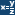.
As you enter expressions into each cell, SAS Decision Manager displays the rule conditions and actions, including the operators and term names that are added by SAS Decision Manager, on the Rule Details tab. For example, suppose you enter the following rule in the editor: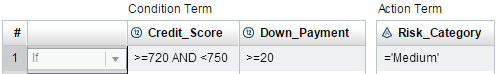SAS Decision Manager displays the following expressions on the Rule Details tab.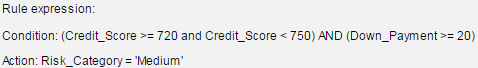For more information about entering expressions, see the following topics:
4. Clickto save the rule set. SAS Decision Manager validates the syntax of the expressions. If it does not detect any problems, it saves the rule set. See Validate the Expressions in a Rule Set for more information.

## Controlling Which Conditions Are Evaluated

You add conditional processing within a rule set by using the IF, ELSE, and OR operators. By default, rules are assigned the keyword IF, which means that the rule’s condition is evaluated regardless of the results of previous rules. You can change this outcome by changing the operator for a rule to ELSE or OR.
If you set a rule’s operator to ELSE, then the rule’s condition is evaluated only if the previous rule’s condition evaluated to false. For example, given the rule set shown the following display, if Order_Quanitity is 12, the condition for rules 1 and 2 evaluates to false, and the condition for rule 3 evaluates to true. Therefore, the action for rule 3 is executed. The conditions for rules 4 and 5 are not evaluated.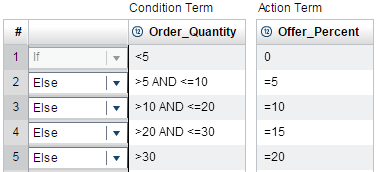Use the OR operator to break up very long condition expressions into multiple condition expressions or to execute the same action expression for each of the several conditions. If you assign the OR operator to a rule, then you cannot enter an action expression for the rule. If any of the conditions evaluate to true, SAS Decision Manager executes the action of the last rule that was assigned the IF or ELSE operator. When you have several consecutive rules that are all assigned the OR operator, only the action for the first rule whose condition evaluates to true is executed. The conditions for the remaining consecutive OR rules are not evaluated.
For example, in the following rule set, rules 1 through 4 all use the action expression that is defined for rule 1. Rules 5 through 8 all use the action expression that is defined for rule 5.
Rule Set That Defines Eight Rules in Two IF Blocks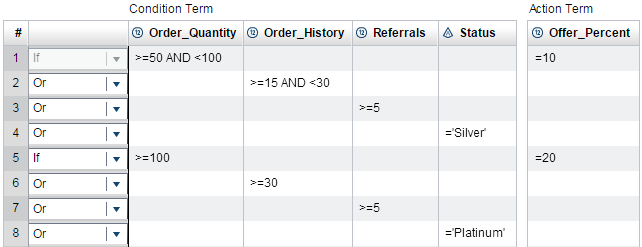An IF block is a series of rules that begins with an IF operator and extends up to but does not include the next IF operator. In the rule set shown in Rule Set That Defines Eight Rules in Two IF Blocks, rules 1 to 4 are an IF block and rules 5 to 8 are a second IF block.

## Tips for Entering Expressions

• A rule that does not have a condition expression always executes.
• A rule that does not have a condition expression must be the last rule in an IF block.
• If you assign the IF operator and a condition expression to a rule but that rule does not have an action expression, the condition expression is evaluated, but no action is taken. (See Controlling Which Conditions Are Evaluated for information about the IF operator.)

## Using the Equation Editor

### Open the Equation Editor

To open the Equation Editor, click in a cell in the rule set editor, and click.
Note: The Lookup tab is available only for condition terms, and the Lookup Value tab is available only for action terms. Buttons for some of the operators might be disabled depending on the data type of the term, and because action expressions can be assignment expressions only.
Expression Tab for a Condition Term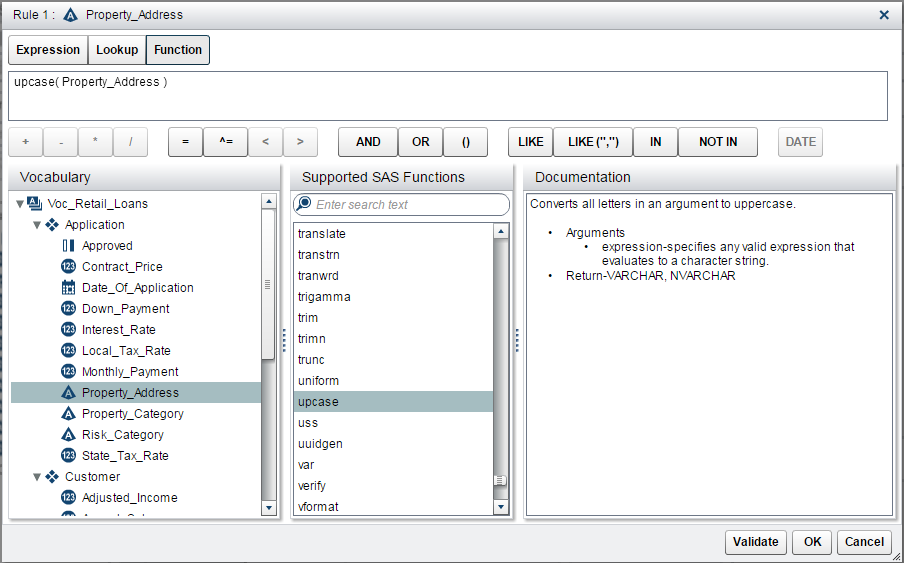### Build an Expression in the Equation Editor

As you add elements to an expression, the Equation Editor builds the expression in the top field of the Expression tab. Click on the operators, vocabulary terms, and domain values as needed to add them to the expression. To add date constants to the expression, click Date. To add numeric constants or character strings to the expression, enter them directly into the top field. (Remember to use the correct punctuation. .)
To build an expression that uses a DS2 function, click the Function tab. Click on a function name to display information about the syntax for that function. Double-click on a function name to add the function to your expression.
To build an expression that uses the LOOKUP or LOOKUPVALUE functions, click the Lookup or LookupValue tabs. You can enter the LOOKUP function in condition expressions only, and you can enter the LOOKUPVALUE function in action expressions only. Specify the LOOKUPVALUE Function in the Equation Editor for more information.
When you are finished building the expression, click OK. The Equation Editor validates the syntax of the expression. If the validation is successful, the editor adds the expression to the cell in the table from which you opened the editor. You can click Validate at any time to check the syntax of the expression that you are building.

### Specify the LOOKUP Function in the Equation Editor

To use the Equation Editor to enter the LOOKUP function, click the Lookup tab. Double-click on the lookup table name that you want to specify in the function call and click OK.
Lookup Tab in the Equation Editor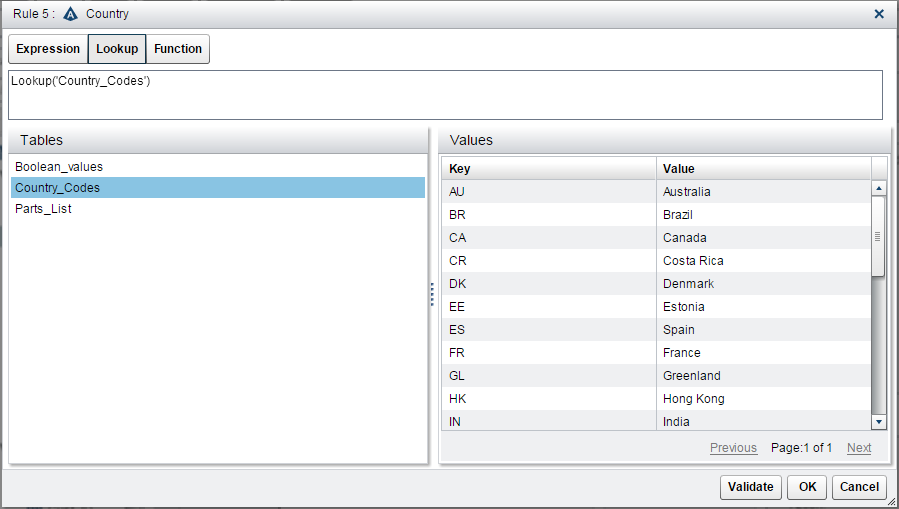For more information, see LOOKUP Function.

### Specify the LOOKUPVALUE Function in the Equation Editor

To use the Equation Editor to enter the LOOKUPVALUE function:
1. Click the LookupValue tab.
2. Double-click on the lookup table name.
3. Specify the term name or the character string that contains the lookup key value. To specify a term, double-click on the term in the Vocabulary column. To specify a character string as the lookup key value, enter the character string in the field at the top of the LookupValue tab. Enclose the string in quotation marks.
4. (Optional) Click Validate to check the syntax of the expression.
5. Click OK.
For more information, see LOOKUPVALUE Function.
LookupValue Tab in the Equation Editor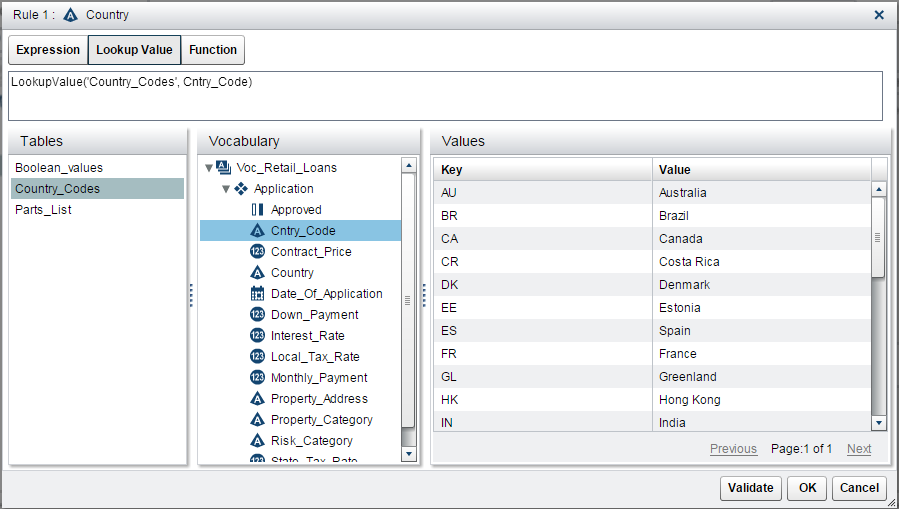## Punctuation for Data Values

Values for some data types might need to be enclosed in quotation marks, as shown in the following table. Date and Datetime values must be followed with `d` and `dt`, respectively.
Punctuation Needed for Data Values
Data Type
Punctuation Needed
Example
Character
Enclose character strings in either single or double quotation marks.
`='Gold Account'`
`="Ineligible"`
`="d'oscail"`
Date
Enter Date values by using the format DDMMMYYYY. Enclose each value in quotation marks followed by `d`.
`='01AUG2015'd`
`>='31AUG2015'd`
Datetime
Enter Datetime values by using the format DDMMMYYYY:HH:MM:SS. Use 24-hour clock notation. Enclose each value in quotation marks followed by `dt`.
`='01AUG2015:15:00:00'dt`
`<='31AUG2015:15:00:00'dt`
Boolean
Boolean values are not enclosed in quotation marks. Enter only `True` or `False`.
`=True`
`=False`

## Operators for Use in Expressions

The following table lists the operators that you can use in an expression. Do not enter a space between the elements of the operators <=, >=, or ^=. Some mnemonic equivalents for these operators cannot be used in SAS Decision Manager expressions. See Operators in Expressions in SAS DS2 Language Reference for more information about the operators shown in the table.
Operators
Operator
Definition
Example
*
Multiply
```0.085 * sales```
/
Divide
```amount / 5```
+
Add
`num + 3`
Subtract
`sale - discount`
=
Equal to
`= maxTriesAllowed`
+value
Leading plus1
+60
^=
Not equal to
```insufficientFunds ^= True```
>
Greater than
```daysLate > 5```
<
Less than
```num < 8```
>=
Greater than or equal to
```balance >= 1000```
<=
Less than or equal to
```balance <= 250```
IN (value-list)
Equal to an item in value-list
`in ('high','medium','low')`
NOT IN (value-list)
Not equal to an item in value-list
`not in (10,20,30)`
LIKE 'pattern-matching-expression'
LIKE ('pattern-matching-expression','pattern-matching-expression')
If the term’s value matches pattern-matching-expression, the result is true.
`like 'HS%PP'`
`like ('_976%','_223%')`
expression AND expression
If both expressions are true, the result is true.
```dateExpired >= '01AUG2015'd AND dateExpired <= '31AUG2015'd```
expression OR expression
If either expression is true, the result is true.
```dateEnrolled >= '01JAN2015' OR member = True```
1The application supports the leading plus (+) operator in action expressions only.

## Using the LIKE Operator

The LIKE operator determines whether the value of a term matches a pattern-matching expression. The syntax of an expression that uses the LIKE operator is as follows:
LIKE 'pattern-matching-expression'
LIKE ('pattern-matching-expression'<,'pattern-matching-expression'>)
If a term’s value matches the pattern that is specified by pattern-matching-expression, the expression evaluates to true (1). Otherwise, the expression evaluates to false (0).
There are three classes of pattern-matching characters.
Pattern-Matching Characters
Character
Description
underscore (_)
Matches any single character
percent sign (%)
Matches any sequence of zero or more characters
Note: Be aware of the effect of trailing blanks. To match values, you might have to use the TRIM function to remove trailing blanks.
any other character
Matches that character
The LIKE expression is case sensitive. To search for mixed-case strings, use the UPCASE function to create an uppercase version of the term that you want to search. You can use a temporary term to store the results of the UPCASE function. (See Step 8 of ) Use the LIKE operator to search the uppercase version of the term.
For example, you can search the term Part_Number for mixed-case strings that begin with HS and end with PP by using the two rules shown in the following display.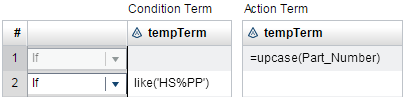The following table shows examples of the matches that result if you search a term that could have these values: Smith, Smooth, Smothers, Smart, Smuggle.
Examples of LIKE Expressions
LIKE Expression Example
Matching Results
`like 'Sm%'`
```Smith, Smooth, Smothers, Smart, Smuggle```
`like '%th'`
`Smith, Smooth`
`like 'S__gg%'`
`Smuggle`
`like 'S_o'`
(no matches)
`like 'S_o%'`
```Smooth, Smothers```
`like 'S%th'`
`Smith, Smooth`

## Using Functions in Expressions

SAS Decision Manager supports the following functions in rule expressions:
• LOOKUP and LOOKUPVALUE functions. See LOOKUP Function and LOOKUPVALUE Function for more information.
• SAS DS2 functions. You can click on a function name in the Equation Editor to display information about the syntax for that function. For additional information about DS2 functions, see SAS DS2 Language Reference.

## Working with Missing Values

You can use the MISSING function to check for missing values. This function returns a 0 (false) or 1 (true). Missing values have a value of `false` when you use them with logical operators such as AND or OR.
For more information, see How DS2 Processes Nulls and SAS Missing Values in SAS DS2 Language Reference.

## Terms and Operators Added by SAS Decision Manager

As you enter expressions into each cell, SAS Decision Manager displays the rule conditions and actions on the Rule Details tab. The operators and term names that are added by SAS Decision Manager are also displayed. Remember these rules when you are entering expressions:
• If you do not specify an operator at the beginning of an expression, SAS Decision Manager adds an equal sign to the beginning of the expression. For example, if you enter `5+x` for an expression, the expression resolves to `=5+x`.
• When an AND or OR operator is followed immediately by another operator in a condition expression, SAS Decision Manager inserts the condition term between the AND or OR operator and the operator that follows it. For example, if you enter ```>5 and <10``` for `myterm`, the expression resolves to `myterm>5 and myterm<10`. SAS Decision Manager inserts the term for top-level AND or OR operators in condition expressions only. It does not insert the term with nested AND or OR operators or in action expressions.

## Leading Plus and Minus Operators

If you specify the leading plus operator in an action expression, SAS Decision Manager adds the term name to the expression. Leading minus operators are not supported.
The condition expression `+1` is invalid. If you enter `+1` as an action expression, the expression resolves to `x=x + 1`. The expression `=+1` is invalid as both a condition and as an action expression.
If you enter `-1` as either a condition or an action expression, the expression is interpreted as a negative number and not as a leading minus operator. The expression resolves to `x=-1`.

## Examples of Expressions

The following table shows examples of expressions that you can specify.
Examples of Expressions
Expression as Entered into the Decision Table for Term X
Resulting Expression
Valid as a Condition Expression
Valid as an Action Expression
`5`
`x=5`
Yes
Yes
`=5`
`x=5`
Yes
Yes
+10
`x=x+10`
No
Yes
–10
`x=–10`
Yes
Yes
`"mystring"`
`x="mystring"`
Yes
Yes
`=term1`
`x=term1`
Yes
Yes
`5 or >100`
`x=5 or x>100`
Yes
No
```^=5 and x<10```
```x^=5 and x<10```
Yes
No
`^=5 or >=(100/4)`
```x^=5 or x>=(100/4)```
Yes
No
`in (10,20,30)`
`x IN (10,20,30)`
Yes
No
`not in ('med','high')`
```x NOT IN ('MED','HIGH')```
Yes
No
```rate in ('med','high')```
```x = rate in ('med','high')```
Yes
Yes
`like ('M77__LL%','MA89_LL%')`
`x LIKE ('M77__LL%','MA89_LL%')`
Yes
No
See Note 1.
`<'10JUN2012'd`
`x<'10JUN2015'd`
Yes
No
`>'10JUN2012:17:00:00'dt`
`x>'10JUN2015:17:00:00'dt`
Yes
No
`=ABS(-10)`
`x=ABS(-10)`
Yes
Yes
`=True`
`x=True`
Yes
Yes
`False`
`x=False`
Yes
Yes
`&myMacroVar`
`x=&myMacroVar`
Yes
Yes
`%EVAL(&myMacroVar)`
`x=%EVAL(&myMacroVar)`
Yes
Yes
`term1=5`
`x=term1=5`
Yes
See Note 3.
Yes
See Note 4.
```term1=3 or term2=5```
```x=term1=3 or term2=5```
Yes
See Note 5.
Yes
See Note 6.
```5 or (x>10 and <20)```
This expression is invalid as both a condition expression and as an action expression. SAS Decision Manager does not add column names after nested AND or OR operators.
`>"mystring"`
This expression is invalid as both a condition expression and as an action expression. SAS Decision Manager checks whether literal types are compatible with the specified operators. Character strings are not compatible with numeric operators.
`=4.6927e-101`
`x=4.6927e-101`
Yes
Yes
Notes:
1. Action expressions must be assignment expressions only.
2. SAS Decision Manager validates macro functions and variables based only on whether the syntax is correct. It does not check to determine whether a macro function or variable will be accessible when the rule set is executed. Macro support in expressions is controlled by a configuration property in SAS Management Console. See Support macros in rule expressions in SAS Decision Manager: Administrator’s Guide for more information.
3. This expression is valid. However, it should be avoided. As a condition statement, this expression checks to determine whether both `x` and `term1` are equal to 5. The recommended way to enter this expression is ```=5 and term1=5```.
4. As an action expression, this expression becomes a Boolean assignment statement. This expression determines whether `term1` is equal to 5, and if so, assigns a value of 1 (true) to `x`. If not, it assigns a value of 0 (false) to `x`.
5. This expression is valid. However, it should be avoided. As a condition statement, this expression checks to determine whether both `x` and `term1` are equal to 3 or whether `term2` is equal to 5. The recommended way to enter this expression is ```(=5 or term1=3) or term2=5```.
6. As an action expression, this expression becomes a Boolean assignment statement. This expression determines whether either `term1` is equal to 3 or `term2` is equal to 5, and if so, assigns a value of 1 (true) to `x`. If not, it assigns a value of 0 (false) to `x`.
7. Leading plus (+) operators are valid in action expressions only. Leading minus (–) operators are not supported. See Leading Plus and Minus Operators for more information.
8. As an action expression, this expression becomes a Boolean assignment statement. The expression determines whether `rate` is equal to `high` or `low`, and if so, assigns a value of 1 (true) to `x`. If not, it assigns a value of 0 (false) to `x`.
9. This expression is valid. However, you should use caution when testing for equality by using scientific notation. Two numbers that appear to be the same might evaluate to different numbers because of the precision involved in scientific notation.
Last updated: February 22, 2017